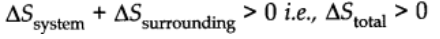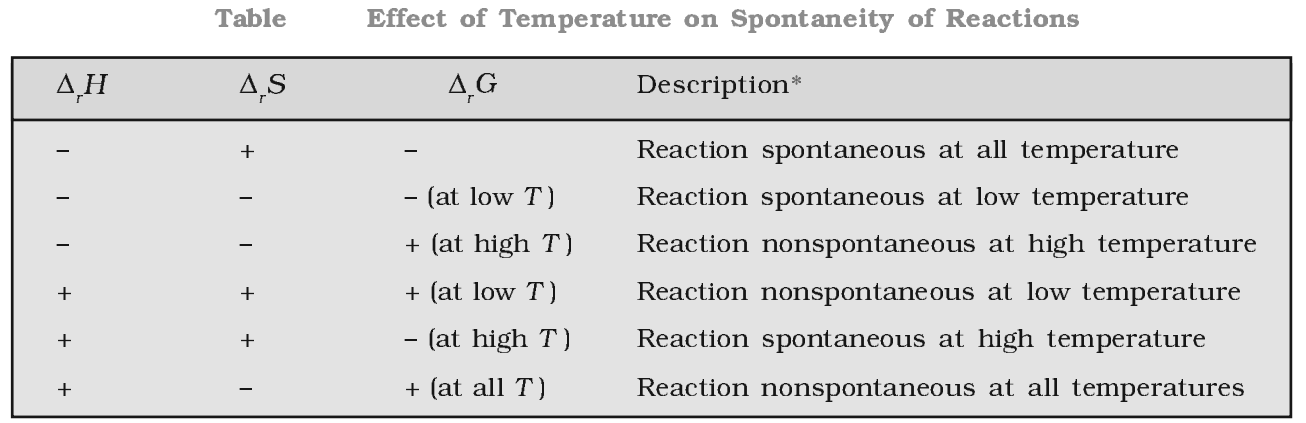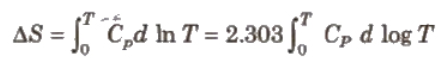# Second Law of Thermodynamics

The entropy of the universe is always increasing in the course of every spontaneous or natural change.

(All spontaneous processes or natural change are thermodynamically irreversible without the help of an external work, i.e., heat cannot flow itself from a colder to hotter body.)# Gibb’s Free Energy

Gibb’s Free energy function gives us a very convenient parameter to judge the spontaneity of a process from system’s perspective.

At a constant temperature and pressure, ∆G = –T∆STOTAL and for a process to be spontaneous, ∆G < 0. The change in Gibb’s free energy can also be represented in terms of the system parameters as:

Mathematically,

G = H – T.S

Change in Gibb’s energy during the process 1S given by Gibb’s Helmholtz equation.

(∆G = G2 -G1 = ∆H – T∆S)

Where, ∆G = Gibb’s free energy

H = enthalpy of system

TS = random energy

∆Gsystem = -T∆Stotal

The Gibb’s energy criterion of spontaneity

∆G > 0, process is non-spontaneity

∆G < 0, process is spontaneous

∆G = 0, process is in equilibrium state# Third Law of Thermodynamics

This law was formulated by Nernst in 1906. According to this law, “The entropy of a perfectly crystalline substance at zero K or absolute zero is taken to be zero.” We can find absolute entropies of pure substances at different temperature.Where, Cp = heat capacities

T = temperature between 0 K and T K

This law is only applicable for perfectly crystalline substance. If there is imperfection at 0 K, the entropy will be large then zero.